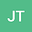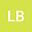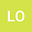A nonlinear elliptic system with a transport term and singular data
•••• Jose Ignacio Tello,
• Lucio Boccardo,
• Luigi Orsina
Jose Ignacio Tello
Universidad Nacional de Educación a Distancia

Corresponding Author:[email protected]

Author ProfileLucio Boccardo
Istituto Lombardo
Author ProfileLuigi Orsina
Sapienza Università di Roma
Author Profile## Abstract

We consider the nonlinear elliptic system { u ∈ W 0 N N − 1 ( Ω ): − div ( M ( x ) ∇ u )+ u = − div ( u M ( x ) ∇ ψ )+ f ( x ) , ψ ∈ W 0 1 , 2 ( Ω ): − div ( M ( x ) ∇ ψ )+ ψ = R ( u )+ E ( x ) ∇ ψ , whereis a bounded, open subset of R N , N≥3 ; M( x) is a coercive, symmetric matrix with L ∞ ( Ω ) coefficients; f( x) and E( x) belong to some Lebesgue space, and R( s) is a continuous function such that 0 ≤ R ( s ) ≤ | s | θ , for θ < 2 N . Using a duality technique, we prove existence of at least a weak solution ( u,ψ) . Moreover, if N=3 or N=4 , we prove under stronger assumptions on f( x) and E( x) that the solution u belongs to W 0 1 , 2 ( Ω ) .